# C++11 sort benchmark

## Posted on October 24, 2012 by Paul

The code for this post is on GitHub: https://github.com/sol-prog/Sort_benchmark.

A good sorting routine is a building block for many numerical algorithms and, because of this, most modern programming languages provide one or more sorting algorithms in their standard library. C++ is not an exception. What varies, from one C++ implementation to another, is the performance of the sorting algorithm.

C++11 lets you use three sorting functions std::qsort, std::sort and std::stable_sort. While std::qsort comes from the C roots of C++, std::sort and std::stable_sort are provided by C++’s Standard Template Library. The new C++11 standard requires that the complexity of std::sort to be $$O(n \cdot \log (n))$$ in the worst case. Historically, in the C++98 standard std::sort was defined as $$O(n \cdot \log (n))$$ on average, with a possible worst case scenario of $$O(n^2)$$.

Today, we have a number of C++ compilers that implements, at least partially, the C++11 standard and I think it could be an interesting experiment to test their performance with a reasonably large data set. For this experiment, we are going to use three compilers Clang 3.2svn with libc++, GCC 4.7.2 with libstc++ and Visual Studio 2012. The test machine is a quad-core Intel i7 Q740 processor with 16GB of RAM. GCC and Clang were compiled from sources on Ubuntu 12.04.1 64 bits and Visual Studio 2012 was installed on Windows 8 Pro 64 bits.

The experiment will consist in measuring the execution time of sorting a double precision array, V, of size N with all the available sorting routines from a standard C++ compiler: std::sort, std::stable_sort and std::qsort. The C++11 standard also defines the chrono library that could theoretically be used for accurate time measurements. In practice, however, at the time of this writing, the only compiler that fully implements std::chrono::steady_clock() is Clang. In order to use the same measurement technique with all three test compilers, we are going to use the Boost 1.51 implementation of the steady_clock.

The size N of the test array, V, will vary from two to one million random numbers on the unit interval. Let’s start by defining four tests:

1. V is filled with unordered random data that will be sorted.
2. V is filled with ordered random data after the first test. We are going to check how much time a sorting algorithm will take to process a sorted input.
3. Start by reversing the data from V and sort the reversed array. We are going to check how much time a sorting algorithm will take to process an array filled with data in decreasing order.
4. Fill V with a unique, random value, and check the performance of the sorting algorithms.

We’ll start by implementing a function that will fill an array with random numbers:

Next, we’ll need a function for sorting the data with std::sort:

The code that tests std::stable_sort is similar with the above function (see the Github repository for this project).

std::qsort needs a helper function that will compare two elements:

and now the actual code that tests std::qsort:

As I’ve said before, the size N of the array V will be increased from two to one million elements, each of the four proposed tests will be repeated for the available sorting algorithms. Each particular test will be repeated one hundred times and the averaged results, with their standard deviation error bars, will be plotted.

In the next three figures, we present the performance of std::sort for the three compilers, for each of the four input cases: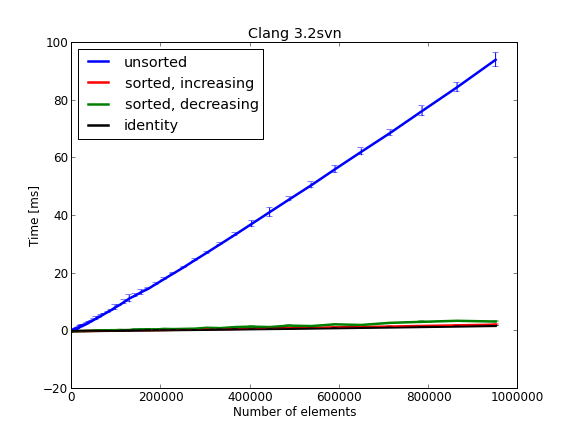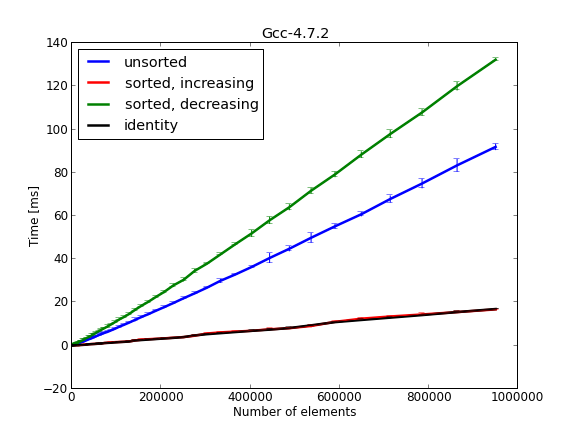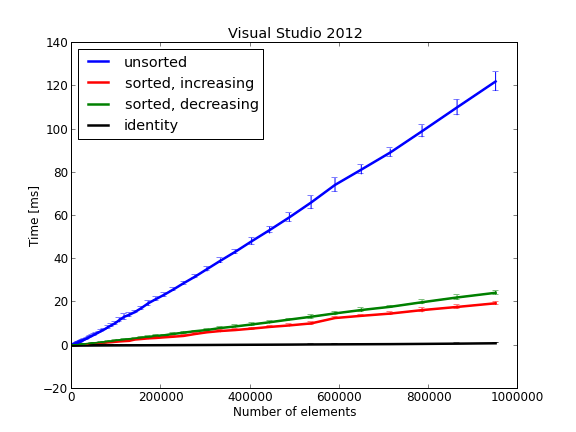From the three compilers used for this experiment, Clang seems to have better performance for std::sort with less than 100 milliseconds processing time for about one million random numbers. Clang also has better results than the other two compilers for sorting already sorted data, less than 10 milliseconds. Visual Studio has similar results. GCC, on the other hand, has problems sorting the reversed array.

Next set of results is for std::stable_sort. For this algorithm we have similar results for all three compilers. Notably, GCC performs much better than for std::sort.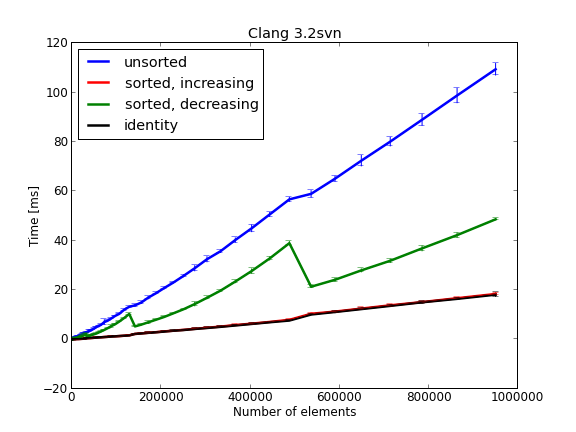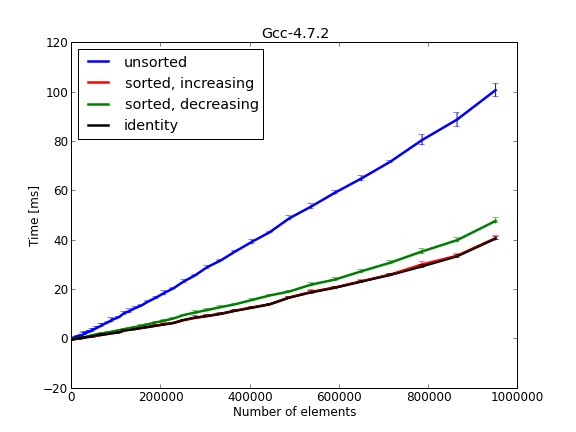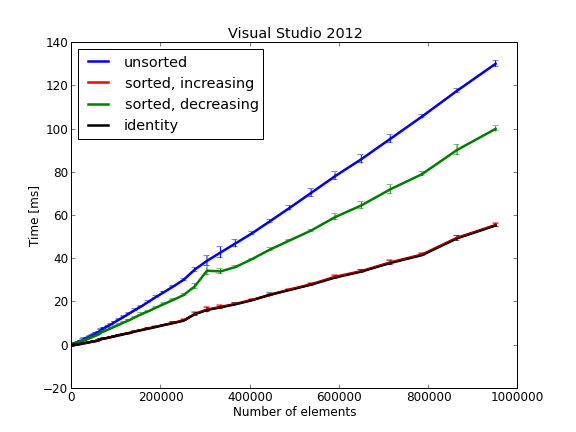The last set of results for std::qsort is presented in the next three figures: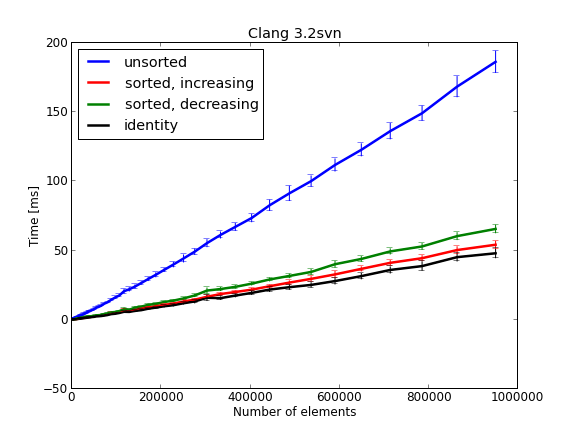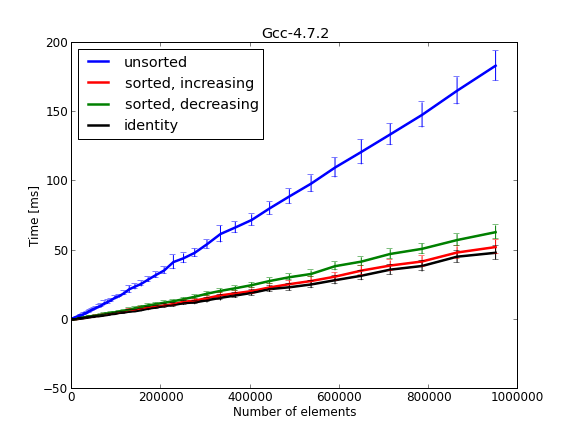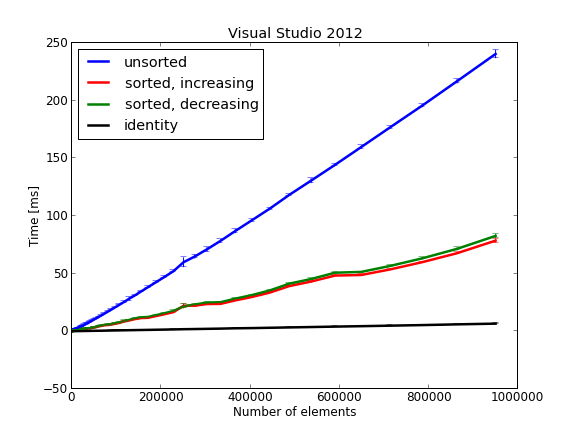For std::qsort, Clang and GCC have similar performance, while Visual Studio has a slightly worse time.

In the next table, we present the size in bytes of the executable generated by each compiler for each algorithm:

 Algorithm Compiler Clang GCC Visual Studio std::sort 24758 34924 28672 std::stable_sort 24758 31086 32768 std::qsort 24758 30670 28672

If you are interested in learning more about the new C++11 syntax I would recommend reading The C++ Programming Language by Bjarne Stroustrup.or, Professional C++ by M. Gregoire, N. A. Solter, S. J. Kleper: